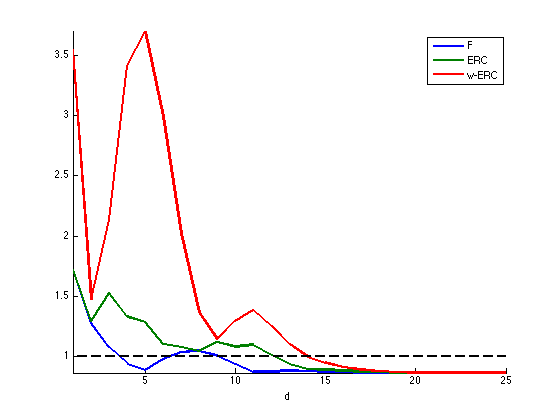$\newcommand{\NN}{\mathbb{N}} \newcommand{\CC}{\mathbb{C}} \newcommand{\GG}{\mathbb{G}} \newcommand{\LL}{\mathbb{L}} \newcommand{\PP}{\mathbb{P}} \newcommand{\QQ}{\mathbb{Q}} \newcommand{\RR}{\mathbb{R}} \newcommand{\VV}{\mathbb{V}} \newcommand{\ZZ}{\mathbb{Z}} \newcommand{\FF}{\mathbb{F}} \newcommand{\KK}{\mathbb{K}} \newcommand{\UU}{\mathbb{U}} \newcommand{\EE}{\mathbb{E}} \newcommand{\Aa}{\mathcal{A}} \newcommand{\Bb}{\mathcal{B}} \newcommand{\Cc}{\mathcal{C}} \newcommand{\Dd}{\mathcal{D}} \newcommand{\Ee}{\mathcal{E}} \newcommand{\Ff}{\mathcal{F}} \newcommand{\Gg}{\mathcal{G}} \newcommand{\Hh}{\mathcal{H}} \newcommand{\Ii}{\mathcal{I}} \newcommand{\Jj}{\mathcal{J}} \newcommand{\Kk}{\mathcal{K}} \newcommand{\Ll}{\mathcal{L}} \newcommand{\Mm}{\mathcal{M}} \newcommand{\Nn}{\mathcal{N}} \newcommand{\Oo}{\mathcal{O}} \newcommand{\Pp}{\mathcal{P}} \newcommand{\Qq}{\mathcal{Q}} \newcommand{\Rr}{\mathcal{R}} \newcommand{\Ss}{\mathcal{S}} \newcommand{\Tt}{\mathcal{T}} \newcommand{\Uu}{\mathcal{U}} \newcommand{\Vv}{\mathcal{V}} \newcommand{\Ww}{\mathcal{W}} \newcommand{\Xx}{\mathcal{X}} \newcommand{\Yy}{\mathcal{Y}} \newcommand{\Zz}{\mathcal{Z}} \newcommand{\al}{\alpha} \newcommand{\la}{\lambda} \newcommand{\ga}{\gamma} \newcommand{\Ga}{\Gamma} \newcommand{\La}{\Lambda} \newcommand{\Si}{\Sigma} \newcommand{\si}{\sigma} \newcommand{\be}{\beta} \newcommand{\de}{\delta} \newcommand{\De}{\Delta} \renewcommand{\phi}{\varphi} \renewcommand{\th}{\theta} \newcommand{\om}{\omega} \newcommand{\Om}{\Omega} \renewcommand{\epsilon}{\varepsilon} \newcommand{\Calpha}{\mathrm{C}^\al} \newcommand{\Cbeta}{\mathrm{C}^\be} \newcommand{\Cal}{\text{C}^\al} \newcommand{\Cdeux}{\text{C}^{2}} \newcommand{\Cun}{\text{C}^{1}} \newcommand{\Calt}{\text{C}^{#1}} \newcommand{\lun}{\ell^1} \newcommand{\ldeux}{\ell^2} \newcommand{\linf}{\ell^\infty} \newcommand{\ldeuxj}{{\ldeux_j}} \newcommand{\Lun}{\text{\upshape L}^1} \newcommand{\Ldeux}{\text{\upshape L}^2} \newcommand{\Lp}{\text{\upshape L}^p} \newcommand{\Lq}{\text{\upshape L}^q} \newcommand{\Linf}{\text{\upshape L}^\infty} \newcommand{\lzero}{\ell^0} \newcommand{\lp}{\ell^p} \renewcommand{\d}{\ins{d}} \newcommand{\Grad}{\text{Grad}} \newcommand{\grad}{\text{grad}} \renewcommand{\div}{\text{div}} \newcommand{\diag}{\text{diag}} \newcommand{\pd}{ \frac{ \partial #1}{\partial #2} } \newcommand{\pdd}{ \frac{ \partial^2 #1}{\partial #2^2} } \newcommand{\dotp}{\langle #1,\,#2\rangle} \newcommand{\norm}{|\!| #1 |\!|} \newcommand{\normi}{\norm{#1}_{\infty}} \newcommand{\normu}{\norm{#1}_{1}} \newcommand{\normz}{\norm{#1}_{0}} \newcommand{\abs}{\vert #1 \vert} \newcommand{\argmin}{\text{argmin}} \newcommand{\argmax}{\text{argmax}} \newcommand{\uargmin}{\underset{#1}{\argmin}\;} \newcommand{\uargmax}{\underset{#1}{\argmax}\;} \newcommand{\umin}{\underset{#1}{\min}\;} \newcommand{\umax}{\underset{#1}{\max}\;} \newcommand{\pa}{\left( #1 \right)} \newcommand{\choice}{ \left\{ \begin{array}{l} #1 \end{array} \right. } \newcommand{\enscond}{ \left\{ #1 \;:\; #2 \right\} } \newcommand{\qandq}{ \quad \text{and} \quad } \newcommand{\qqandqq}{ \qquad \text{and} \qquad } \newcommand{\qifq}{ \quad \text{if} \quad } \newcommand{\qqifqq}{ \qquad \text{if} \qquad } \newcommand{\qwhereq}{ \quad \text{where} \quad } \newcommand{\qqwhereqq}{ \qquad \text{where} \qquad } \newcommand{\qwithq}{ \quad \text{with} \quad } \newcommand{\qqwithqq}{ \qquad \text{with} \qquad } \newcommand{\qforq}{ \quad \text{for} \quad } \newcommand{\qqforqq}{ \qquad \text{for} \qquad } \newcommand{\qqsinceqq}{ \qquad \text{since} \qquad } \newcommand{\qsinceq}{ \quad \text{since} \quad } \newcommand{\qarrq}{\quad\Longrightarrow\quad} \newcommand{\qqarrqq}{\quad\Longrightarrow\quad} \newcommand{\qiffq}{\quad\Longleftrightarrow\quad} \newcommand{\qqiffqq}{\qquad\Longleftrightarrow\qquad} \newcommand{\qsubjq}{ \quad \text{subject to} \quad } \newcommand{\qqsubjqq}{ \qquad \text{subject to} \qquad }$

Performance of Sparse Recovery Using L1 Minimization

# Performance of Sparse Recovery Using L1 Minimization

This tour explores theoritical garantees for the performance of recovery using $$\ell^1$$ minimization.

## Installing toolboxes and setting up the path.

You need to download the following files: signal toolbox and general toolbox.

You need to unzip these toolboxes in your working directory, so that you have toolbox_signal and toolbox_general in your directory.

For Scilab user: you must replace the Matlab comment '%' by its Scilab counterpart '//'.

Recommandation: You should create a text file named for instance numericaltour.sce (in Scilab) or numericaltour.m (in Matlab) to write all the Scilab/Matlab command you want to execute. Then, simply run exec('numericaltour.sce'); (in Scilab) or numericaltour; (in Matlab) to run the commands.

Execute this line only if you are using Matlab.

getd = @(p)path(p,path); % scilab users must *not* execute this


Then you can add the toolboxes to the path.

getd('toolbox_signal/');
getd('toolbox_general/');


## Sparse $$\ell^1$$ Recovery

We consider the inverse problem of estimating an unknown signal $$x_0 \in \RR^N$$ from noisy measurements $$y=\Phi x_0 + w \in \RR^P$$ where $$\Phi \in \RR^{P \times N}$$ is a measurement matrix with $$P \leq N$$, and $$w$$ is some noise.

This tour is focused on recovery using $$\ell^1$$ minimization $x^\star \in \uargmin{x \in \RR^N} \frac{1}{2}\norm{y-\Phi x}^2 + \la \normu{x}.$

Where there is no noise, we consider the problem $$\Pp(y)$$ $x^\star \in \uargmin{\Phi x = y} \normu{x}.$

We are not concerned here about the actual way to solve this convex problem (see the other numerical tours on sparse regularization) but rather on the theoritical analysis of wether $$x^\star$$ is close to $$x_0$$.

More precisely, we consider the following three key properties

• Noiseless identifiability: $$x_0$$ is the unique solution of $$\Pp(y)$$ for $$y=\Phi x_0$$.
• Robustess to small noise:: one has $$\norm{x^\star - x_0} = O(\norm{w})$$ for $$y=\Phi x_0+w$$ if $$\norm{w}$$ is smaller than an arbitrary small constant that depends on $$x_0$$ if $$\la$$ is well chosen according to $$\norm{w}$$.
• Robustess to bounded noise: same as above, but $$\norm{w}$$ can be arbitrary.

Note that noise robustness implies identifiability, but the converse is not true in general.

## Coherence Criteria

The simplest criteria for identifiality are based on the coherence of the matrix $$\Phi$$ and depends only on the sparsity $$\norm{x_0}_0$$ of the original signal. This criteria is thus not very precise and gives very pessimistic bounds.

The coherence of the matrix $$\Phi = ( \phi_i )_{i=1}^N \in \RR^{P \times N}$$ with unit norm colum $$\norm{\phi_i}=1$$ is $\mu(\Phi) = \umax{i \neq j} \abs{\dotp{\phi_i}{\phi_j}}.$

Compute the correlation matrix (remove the diagonal of 1's).

remove_diag = @(C)C-diag(diag(C));
Correlation = @(Phi)remove_diag(abs(Phi'*Phi));


Compute the coherence $$\mu(\Phi)$$.

maxall = @(C)max(C(:));
mu = @(Phi)maxall(Correlation(Phi));


The condition $\normz{x_0} < \frac{1}{2}\pa{1 + \frac{1}{\mu(\Phi)}}$ implies that $$x_0$$ is identifiable, and also implies to robustess to small and bounded noise.

Equivalently, this condition can be written as $$\text{Coh}(\normz{x_0})<1$$ where $\text{Coh}(k) = \frac{k \mu(\Phi)}{ 1 - (k-1)\mu(\Phi) }$

Coh = @(Phi,k)(k * mu(Phi)) / ( 1 - (k-1) * mu(Phi) );


Generate a matrix with random unit columns in $$\RR^P$$.

normalize = @(Phi) Phi ./ repmat(sqrt(sum(Phi.^2)), [size(Phi,1) 1]);
PhiRand = @(P,N)normalize(randn(P,N));
Phi = PhiRand(250,1000);


Compute the coherence and the maximum possible sparsity to ensure recovery using the coherence bound.

c = mu(Phi);
fprintf('Coherence:    %.2f\n', c);
fprintf('Sparsity max: %d\n', floor(1/2*(1+1/c)) );

Coherence:    0.30
Sparsity max: 2


Exercice 1: (check the solution) Display how the average coherence of a random matrix decays with the redundancy $$\eta = P/N$$ of the matrix $$\Phi$$. Can you derive an empirical law between $$P$$ and the maximal sparsity?

exo1;## Support and Sign-based Criteria

In the following we will consider the support $\text{supp}(x_0) = \enscond{i}{x_0(i) \neq 0}$ of the vector $$x_0$$. The co-support is its complementary $$I^c$$.

supp   = @(s)find(abs(s)>1e-5);
cosupp = @(s)find(abs(s)<1e-5);


Given some support $$I \subset \{0,\ldots,N-1\}$$, we will denote as $$\Phi = (\phi_m)_{m \in I} \in \RR^{N \times \abs{I}}$$ the sub-matrix extracted from $$\Phi$$ using the columns indexed by $$I$$.

J.J. Fuchs introduces a criteria $$F$$ for identifiability that depends on the sign of $$x_0$$.

J.J. Fuchs. Recovery of exact sparse representations in the presence of bounded noise. IEEE Trans. Inform. Theory, 51(10), p. 3601-3608, 2005

Under the condition that $$\Phi_I$$ has full rank, the $$F$$ measure of a sign vector $$s \in \{+1,0,-1\}^N$$ with $$\text{supp}(s)=I$$ reads $\text{F}(s) = \norm{ \Psi_I s_I }_\infty \qwhereq \Psi_I = \Phi_{I^c}^* \Phi_I^{+,*}$ where $$A^+ = (A^* A)^{-1} A^*$$ is the pseudo inverse of a matrix $$A$$.

The condition $\text{F}(\text{sign}(x_0))<1$ implies that $$x_0$$ is identifiable, and also implies to robustess to small noise. It does not however imply robustess to a bounded noise.

Compute $$\Psi_I$$ matrix.

PsiI = @(Phi,I)Phi(:, setdiff(1:size(Phi,2),I) )' * pinv(Phi(:,I))';


Compute $$\text{F}(s)$$.

F = @(Phi,s)norm(PsiI(Phi,supp(s))*s(supp(s)), 'inf');


The Exact Recovery Criterion (ERC) of a support $$I$$, introduced by Tropp in

J. A. Tropp. Just relax: Convex programming methods for identifying sparse signals. IEEE Trans. Inform. Theory, vol. 52, num. 3, pp. 1030-1051, Mar. 2006.

Under the condition that $$\Phi_I$$ has full rank, this condition reads $\text{ERC}(I) = \norm{\Psi_{I}}_{\infty,\infty} = \umax{j \in I^c} \norm{ \Phi_I^+ \phi_j }_1.$ where $$\norm{A}_{\infty,\infty}$$ is the $$\ell^\infty-\ell^\infty$$ operator norm of a matrix $$A$$, computed with the Matlab command norm(A,'inf').

erc = @(Phi,I)norm(PsiI(Phi,I), 'inf');


The condition $\text{ERC}(\text{supp}(x_0))<1$ implies that $$x_0$$ is identifiable, and also implies to robustess to small and bounded noise.

One can prove that the ERC is the maximum of the F criterion for all signs of the given support $\text{ERC}(I) = \umax{ s, \text{supp}(s) \subset I } \text{F}(s).$

The weak-ERC is an approximation of the ERC using only the correlation matrix $\text{w-ERC}(I) = \frac{ \umax{j \in I^c} \sum_{i \in I} \abs{\dotp{\phi_i}{\phi_j}} }{ 1-\umax{j \in I} \sum_{i \neq j \in I} \abs{\dotp{\phi_i}{\phi_j}} }$

g = @(C,I)sum(C(:,I),2);
werc_g = @(g,I,J)max(g(J)) / (1-max(g(I)));
werc = @(Phi,I)werc_g( g(Correlation(Phi),I), I, setdiff(1:size(Phi,2),I) );


One has, if $$\text{w-ERC}(I)>0$$, for $$I = \text{supp}(s)$$, $\text{F}(s) \leq \text{ERC}(I) \leq \text{w-ERC}(I) \leq \text{Coh}(\abs{I}).$

This shows in particular that the condition $\text{w-ERC}(\text{supp}(x_0))<1$ implies identifiability and robustess to small and bounded noise.

Exercice 2: (check the solution) Show that this inequality holds on a given matrix. What can you conclude about the sharpness of these criteria ?

exo2;

N=2000, P=1990, |I|=6
F(s)    =0.21
ERC(I)  =0.27
w-ERC(s)=0.30
Coh(|s|)=1.72


Exercice 3: (check the solution) For a given matrix $$\Phi$$ generated using PhiRand, draw as a function of the sparsity $$k$$ the probability that a random sign vector $$s$$ of sparsity $$\norm{s}_0=k$$ satisfies the conditions $$\text{F}(x_0)<1$$, $$\text{ERC}(x_0)<1$$ and $$\text{w-ERC}(x_0)<1$$

exo3;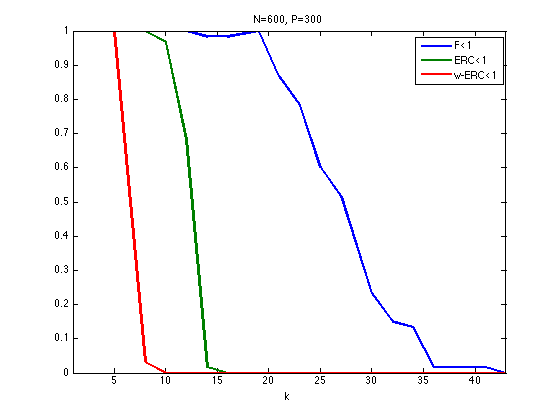## Restricted Isometry Criteria

The restricted isometry constants $$\de_k^1,\de_k^2$$ of a matrix $$\Phi$$ are the smallest $$\de^1,\de^2$$ that satisfy $\forall x \in \RR^N, \quad \norm{x}_0 \leq k \qarrq (1-\de^1)\norm{x}^2 \leq \norm{\Phi x}^2 \leq (1+\de^2)\norm{x}^2.$

E. Candes shows in

E. J. Candès. The restricted isometry property and its implications for compressed sensing. Compte Rendus de l'Academie des Sciences, Paris, Serie I, 346 589-592

that if $\de_{2k} \leq \sqrt{2}-1 ,$ then $$\norm{x_0} \leq k$$ implies identifiability as well as robustness to small and bounded noise.

The stability constant $$\la^1(A), \la^2(A)$$ of a matrix $$A = \Phi_I$$ extracted from $$\Phi$$ is the smallest $$\tilde \la_1,\tilde \la_2$$ such that $\forall \al \in \RR^{\abs{I}}, \quad (1-\tilde\la_1)\norm{\al}^2 \leq \norm{A \al}^2 \leq (1+\tilde \la_2)\norm{\al}^2.$

These constants $$\la^1(A), \la^2(A)$$ are easily computed from the largest and smallest eigenvalues of $$A^* A \in \RR^{\abs{I} \times \abs{I}}$$

minmax = @(v)deal(1-min(v),max(v)-1);
ric = @(A)minmax(eig(A'*A));


The restricted isometry constant of $$\Phi$$ are computed as the largest stability constants of extracted matrices $\de^\ell_k = \umax{ \abs{I}=k } \la^\ell( \Phi_I ).$

The eigenvalues of $$\Phi$$ are essentially contained in the interval $$[a,b]$$ where $$a=(1-\sqrt{\be})^2$$ and $$b=(1+\sqrt{\be})^2$$ with $$\beta = k/P$$ More precisely, as $$k=\be P$$ tends to infinity, the distribution of the eigenvalues tends to the Marcenko-Pastur law $$f_\be(\la) = \frac{1}{2\pi \be \la}\sqrt{ (\la-b)^+ (a-\la)^+ }.$$

Exercice 4: (check the solution) Display, for an increasing value of $$k$$ the histogram of repartition of the eigenvalues $$A^* A$$ where $$A$$ is a Gaussian matrix of size $$(P,k)$$ and variance $$1/P$$. For this, accumulate the eigenvalues for many realization of $$A$$.

exo4;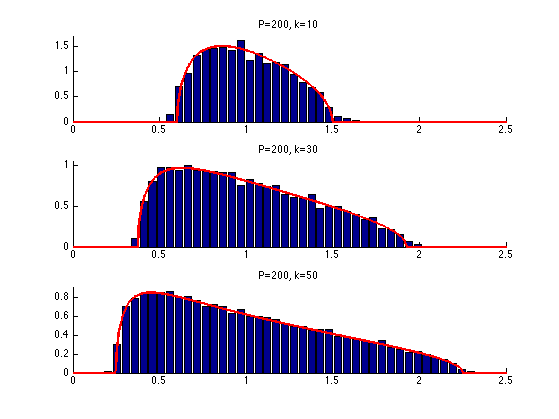Exercice 5: (check the solution) Estimate numerically lower bound on $$\de_k^1,\de_k^2$$ by Monte-Carlo sampling of sub-matrices.

exo5;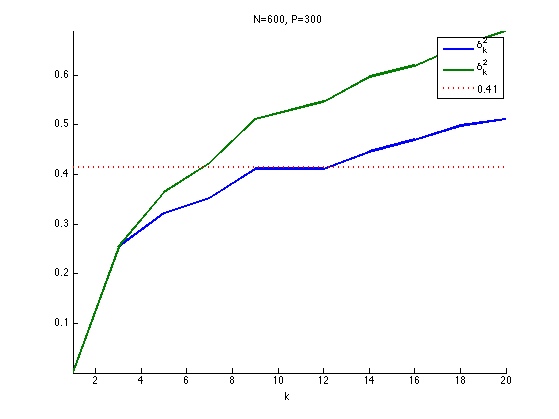## Sparse Spikes Deconvolution

We now consider a convolution dictionary $$\Phi$$. Such a dictionary is used with sparse regulariz

Second derivative of Gaussian kernel $$g$$ with a given variance $$\si^2$$.

sigma = 6;
g = @(x)(1-x.^2/sigma^2).*exp(-x.^2/(2*sigma^2));


Create a matrix $$\Phi$$ so that $$\Phi x = g \star x$$ with periodic boundary conditions.

P = 1024;
[Y,X] = meshgrid(1:P,1:P);
Phi = normalize(g(mod(X-Y+P/2, P)-P/2));


To improve the conditionning of the dictionary, we sub-sample its atoms, so that $$P = \eta N > N$$.

eta = 2;
N = P/eta;
Phi = Phi(:,1:eta:end);


Plot the correlation function associated to the filter. Can you determine the value of the coherence $$\mu(\Phi)$$?

c = Phi'*Phi;
c = abs(c(:,end/2));
clf;
h = plot(c(end/2-50:end/2+50), '.-'); set(h, 'LineWidth', 1);
axis tight;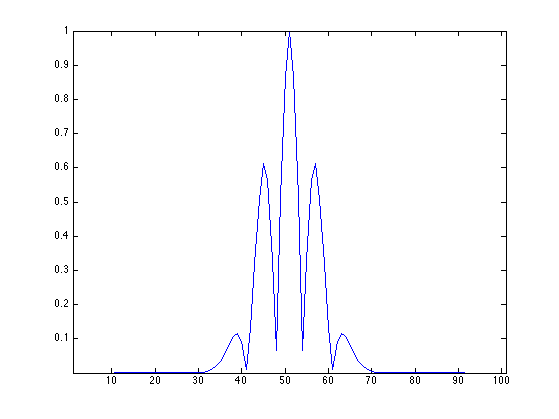Create a data a sparse $$x_0$$ with two diracs of opposite signes with spacing $$d$$.

twosparse = @(d)circshift([1; zeros(d,1); -1; zeros(N-d-2,1)], round(N/2-d/2));


Display $$x_0$$ and $$\Phi x_0$$.

x0 = twosparse(50);
clf;
subplot(2,1,1);
h = plot(x0, 'r'); axis tight;
subplot(2,1,2);
h = plot(Phi*x0, 'b'); axis tight;
set(h, 'LineWidth', 2);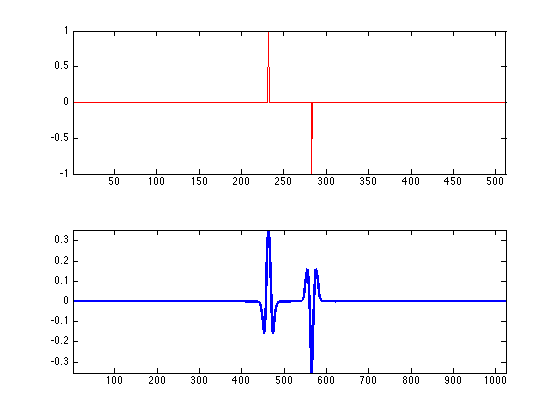Exercice 6: (check the solution) Plot the evolution of the criteria F, ERC and Coh as a function of $$d$$. Do the same plot for other signs patterns for $$x_0$$. Do the same plot for a Dirac comb with a varying spacing $$d$$.

exo6;